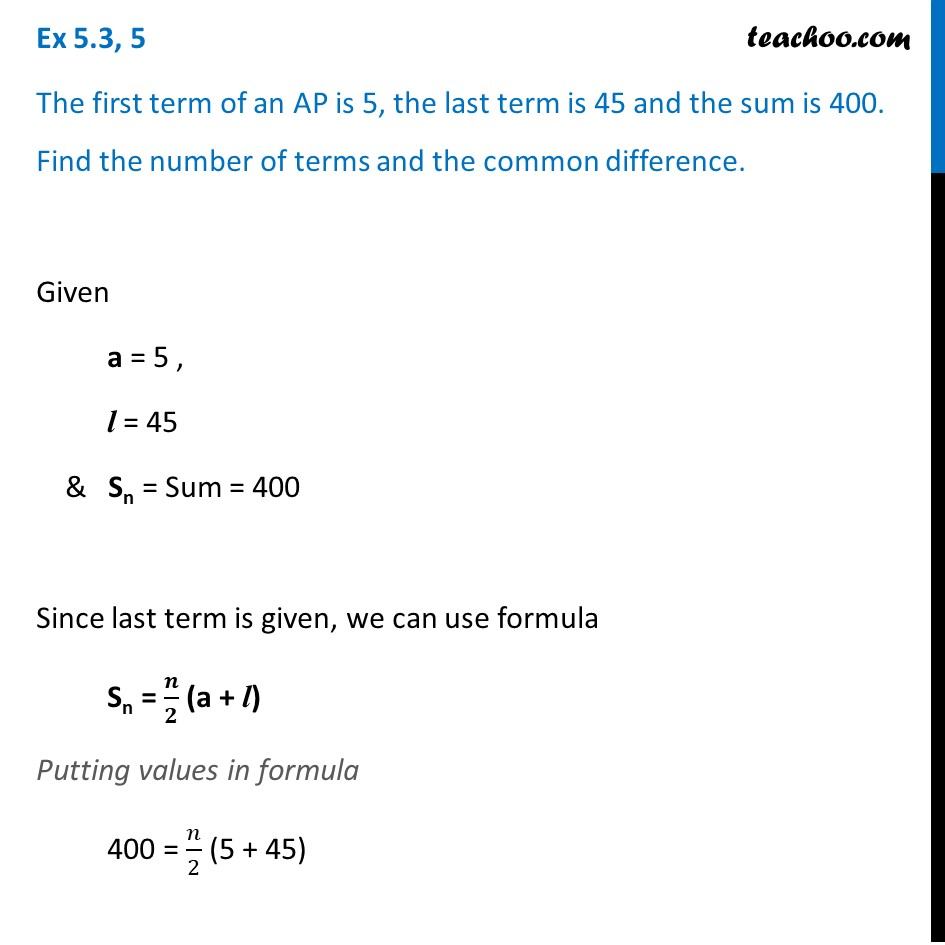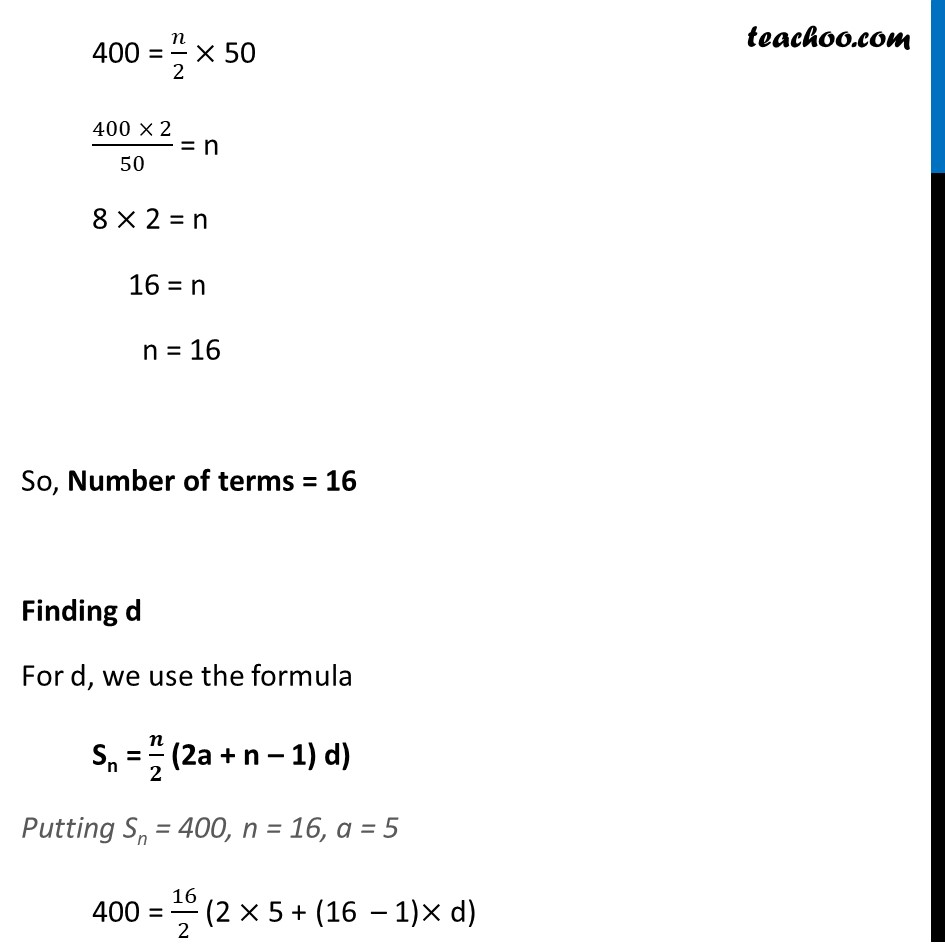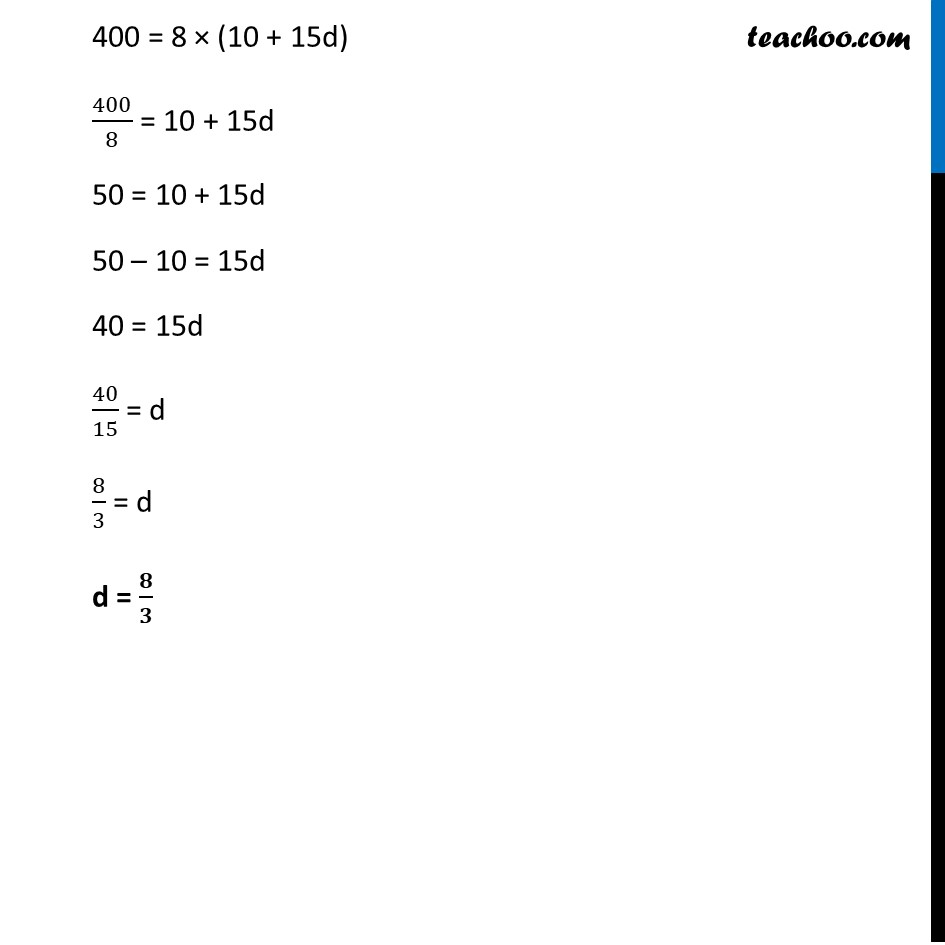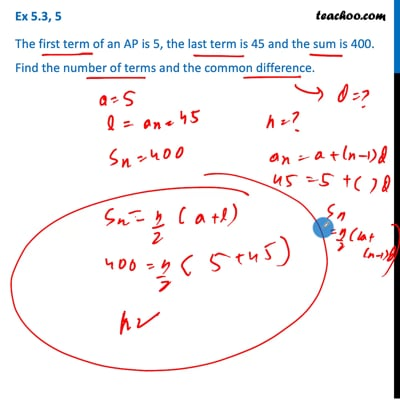Finding number of terms given s

Chapter 5 Class 10 Arithmetic Progressions (Term 2)
Concept wiseThis video is only available for Teachoo black users

### Transcript

Ex 5.3, 5 The first term of an AP is 5, the last term is 45 and the sum is 400. Find the number of terms and the common difference. Given a = 5 , l = 45 & Sn = Sum = 400 Since last term is given, we can use formula Sn = 𝒏/𝟐 (a + l) Putting values in formula 400 = 𝑛/2 (5 + 45) 400 = 𝑛/2 × 50 (400 × 2)/50 = n 8 × 2 = n 16 = n n = 16 So, Number of terms = 16 Finding d For d, we use the formula Sn = 𝒏/𝟐 (2a + n – 1) d) Putting Sn = 400, n = 16, a = 5 400 = 16/2 (2 × 5 + (16 – 1)× d) 400 = 8 × (10 + 15d) 400/8 = 10 + 15d 50 = 10 + 15d 50 – 10 = 15d 40 = 15d 40/15 = d 8/3 = d d = 𝟖/𝟑